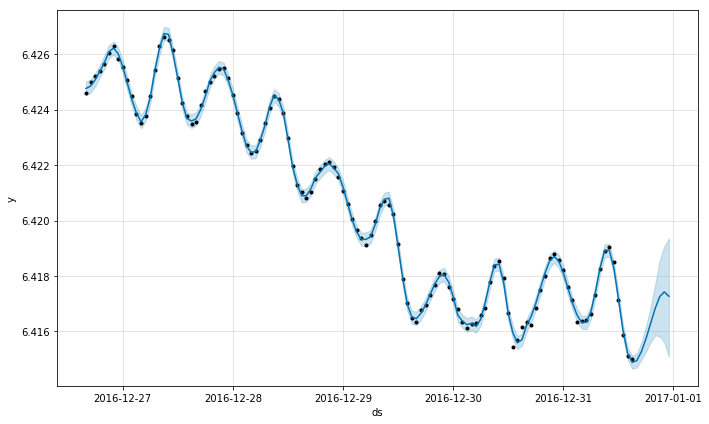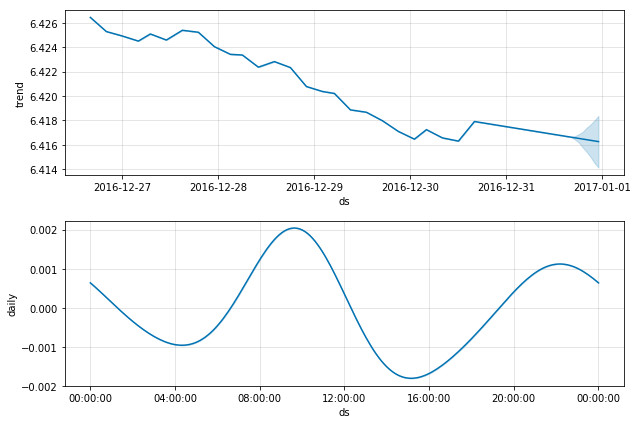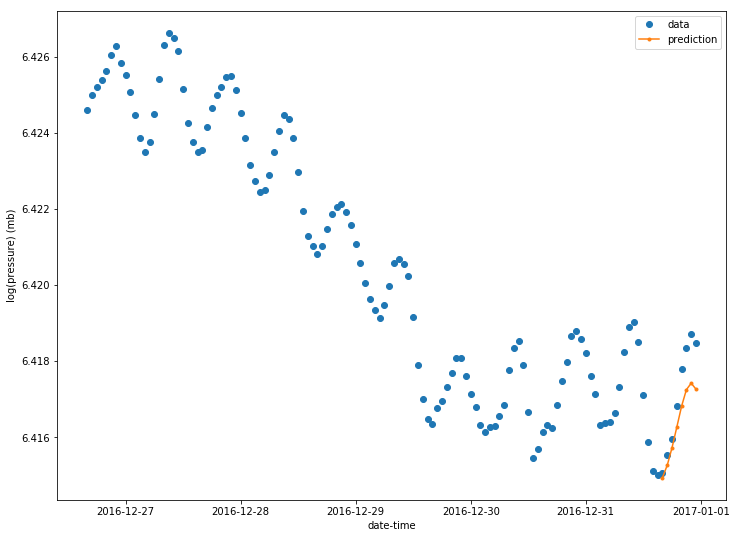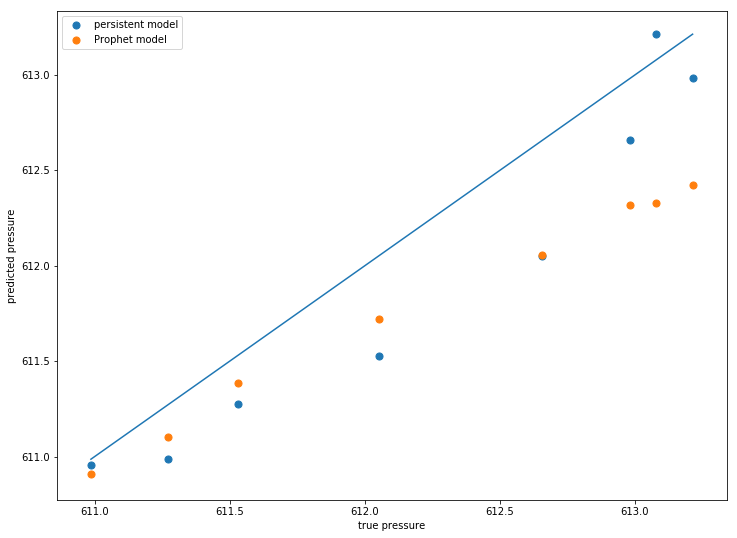## Weather at the Mauna Kea Canadian-France-Hawaii Telescope¶

version 1.0 1/4/2018

Wing-Fai Thi

### Aim of the notebook:¶

• a few hour horizon weather prediction
• use of the Facebook prophet package

### Requirements:¶

• Python 2.7 with numpy, pandas, matplolib, and sklearn packages installed
• first one has to download Prophet and its dependencies (STAN, Cython, Matplotlib).
• the dataset is provided by the Mauna Kea Weather Center. I am usig the data gatered at the CFHT.

### Warning:¶

• The Mauna Kea Weather Center provides much more accurate predictions. The exercise here is intented to show how to use the Facebook Time Series prediciton package and cannot replace actual predictions by professional meteorologists.
In :
import warnings
warnings.filterwarnings('ignore')

In :
import os
import numpy as np
import pandas as pd
import matplotlib.pyplot as plt
from datetime import datetime
from sklearn.externals import joblib # if one wants to save the Phrophet model

In :
# parse the input
def parse(x):
return datetime.strptime(x, '%Y %m %d %H %M') # year month day hour minute

# combine the 4 columns into a Pandas timestamp
# The data are in a space-separated table format
delim_whitespace=True, # white space delimiter
date_parser=parse, # name of the function used to parse the data
parse_dates ={'datetime' : [0,1,2,3,4]})
columns=['datetime','wind speed','wind direction', # assign a column header
'temperature','relative humidity','pressure']
dataset.columns = columns
dataset.dtypes

Out:
datetime             datetime64[ns]
wind speed                    int64
wind direction                int64
temperature                 float64
relative humidity             int64
pressure                    float64
dtype: object
In :
dataset.head()

Out:
datetime wind speed wind direction temperature relative humidity pressure
0 2016-01-01 00:00:00 19 348 2.05 7 618.4
1 2016-01-01 00:01:00 19 351 2.05 7 618.4
2 2016-01-01 00:02:00 18 0 2.05 7 618.4
3 2016-01-01 00:03:00 17 353 2.05 7 618.4
4 2016-01-01 00:04:00 19 346 2.02 7 618.4

The data are recorded every minutes, which is a pretty high rate. Here for our prediction purpose we make a 60 minute averaging of the data.

In :
# make a 1 hr rolling average
# The first column is the datatime and we will not roll it
nb_minutes = 60
avg_dataset = pd.DataFrame.copy(dataset)
avg_dataset.iloc[:,1:5]=avg_dataset.iloc[:,1:5].rolling(nb_minutes).mean()
avg_dataset=avg_dataset.iloc[::nb_minutes,:].dropna()
print avg_dataset.shape

(8757, 6)

In :
# alternative
avg_dataset = pd.DataFrame.copy(dataset) # make first a copy of the original data
avg_dataset = avg_dataset.resample('H',on='datetime').mean() # resample to the hourly-average
print avg_dataset.shape

(8784, 5)

In :
avg_dataset.reset_index(inplace=True) # move the index as a column inplace

Out:
datetime wind speed wind direction temperature relative humidity pressure
0 2016-01-01 00:00:00 25.300000 330.700000 2.544500 6.283333 618.076667
1 2016-01-01 01:00:00 31.650000 272.600000 2.839833 5.516667 617.520000
2 2016-01-01 02:00:00 29.800000 133.983333 2.738000 5.000000 617.263333
3 2016-01-01 03:00:00 28.483333 25.916667 1.624000 5.733333 617.036667
4 2016-01-01 04:00:00 32.766667 154.383333 1.199667 6.516667 617.041667

We will train the model over a few days. One can try models with different training time.

In :
n_train_hours = 5*24 # 1 yr * 24 hrs
horizon = 8
pressure = avg_dataset[['datetime','pressure']]
pressure.columns=['ds','y'] # change the headers for prophet
pressure['y'] = np.log(pressure['y'])
n0 = avg_dataset.shape
n1 = n0 - n_train_hours - horizon
n2 = n0 - horizon
pressure_train = pressure.iloc[n1:n2, :]
pressure_train.shape

Out:
(120, 2)

We first instantiate the Prophet class. The predicted value is yhat with yhat_lower abd yhat_upper as upper and lower possible values. Prophet uses the same API than scikit-learn.

In :
from fbprophet import Prophet
m = Prophet(yearly_seasonality=False,weekly_seasonality=False)
m.fit(pressure_train);

In :
#future = m.make_future_dataframe(periods=horizon,freq='H')
future  = pressure.iloc[n1:,:]
forecast = m.predict(future)
forecast[['ds', 'yhat', 'yhat_lower', 'yhat_upper']].tail()

Out:
ds yhat yhat_lower yhat_upper
123 2016-12-31 19:00:00 6.416273 6.415603 6.416874
124 2016-12-31 20:00:00 6.416823 6.415859 6.417688
125 2016-12-31 21:00:00 6.417256 6.415823 6.418572
126 2016-12-31 22:00:00 6.417426 6.415608 6.419074
127 2016-12-31 23:00:00 6.417269 6.415086 6.419354

m is a Prophet object and can be plotted with predifined settings by Prophet

In :
# Pressure forecast with a 8hr horizon
m.plot(forecast);A time series component decomposition is avaialble. We can clearly see the daily pressure pressure variation. The trend has been a decreasing pressure over the last 7 days.

In :
m.plot_components(forecast);In :
from matplotlib.dates import date2num
t = pressure['ds']
p = pressure['y']
dates  = date2num(t)
datesf = date2num(forecast['ds'])
plt.figure(figsize=(12,9))
plt.plot_date(dates[n1:],p[n1:],marker='o',label='data')
plt.plot_date(datesf[-horizon:],forecast['yhat'][-horizon:],
marker='.',linestyle='-',label='prediction')
plt.legend()
plt.xlabel('date-time')
plt.ylabel('log(pressure) (mb)')
plt.show()The 5-day window for the pressure predictions gives reasonable results.

In :
import math
# compute the mean square error
y_pred = np.array(np.exp(forecast['yhat'][-horizon:]))
y_true = np.array(np.exp(p[-horizon:]))
y_persistent = np.array(np.exp(p[n0-horizon-1:n0-1]))
mse = ((y_pred-y_true)**2).mean()
print('Prediction quality: {:.2f} MSE ({:.2f} RMSE)'.format(mse, math.sqrt(mse)))

Prediction quality: 0.27 MSE (0.52 RMSE)


A persistent model is just using the previous value as a prediction. In our case, the persistent model can predict for the next hour.

In :
mse_persistent = ((y_persistent-y_true)**2).mean()
print('Persistent model quality: {:.2f} MSE ({:.2f} RMSE)'.format(mse_persistent,
math.sqrt(mse_persistent)))

Persistent model quality: 0.12 MSE (0.35 RMSE)

In :
plt.figure(figsize=(12,9))
plt.scatter(y_true,y_persistent,label='persistent model',s=50)
plt.scatter(y_true,y_pred,label='Prophet model',s=50)
plt.plot([y_true.min(),y_true.max()],[y_true.min(),y_true.max()])
plt.xlabel('true pressure')
plt.ylabel('predicted pressure')
plt.legend()
plt.show()An eight-hour horizon should be really a maximum before the predictions become really bad.

In this notebook, we have learnt how to:

• convert values into a pandas datetime format
• use the Facebook Prophet package to make time series predictions

One can try to predict other meteorological data as well like the temperature.